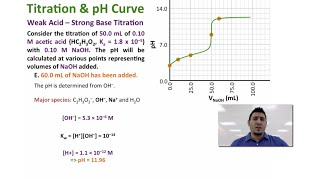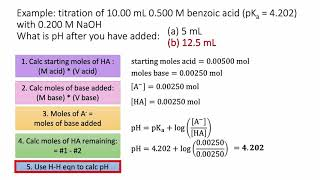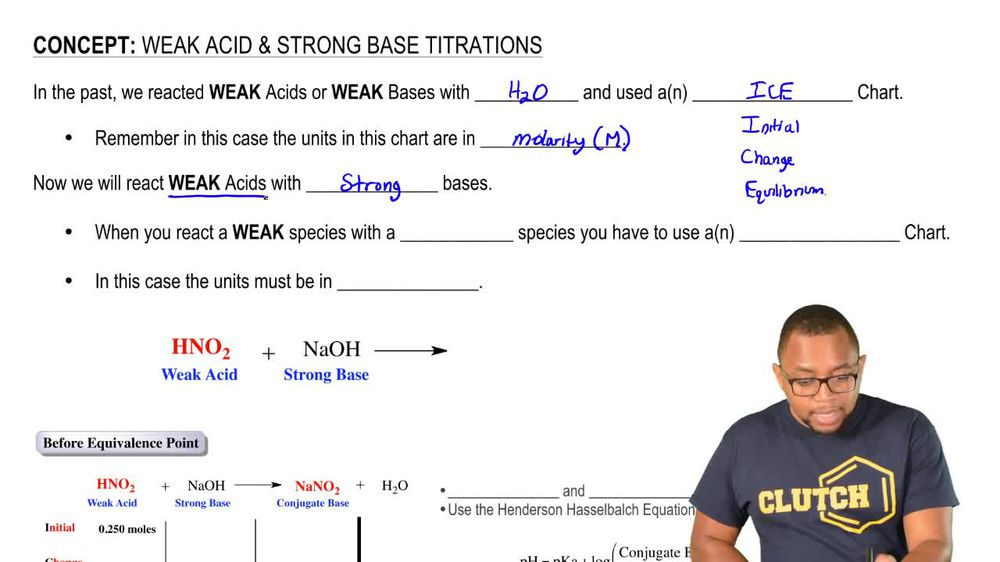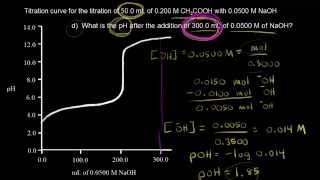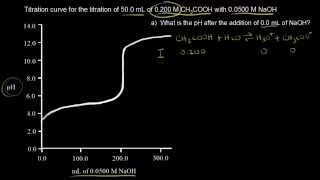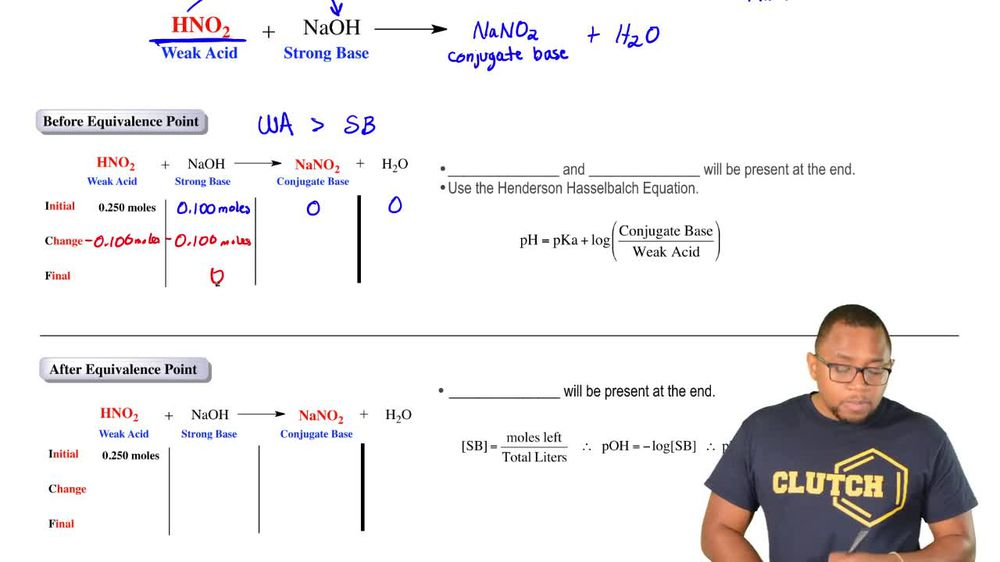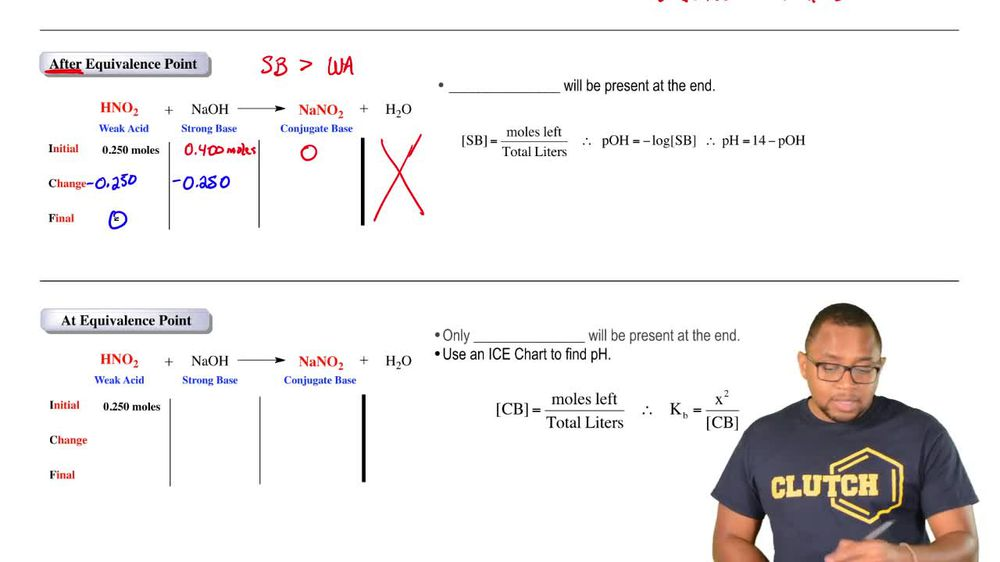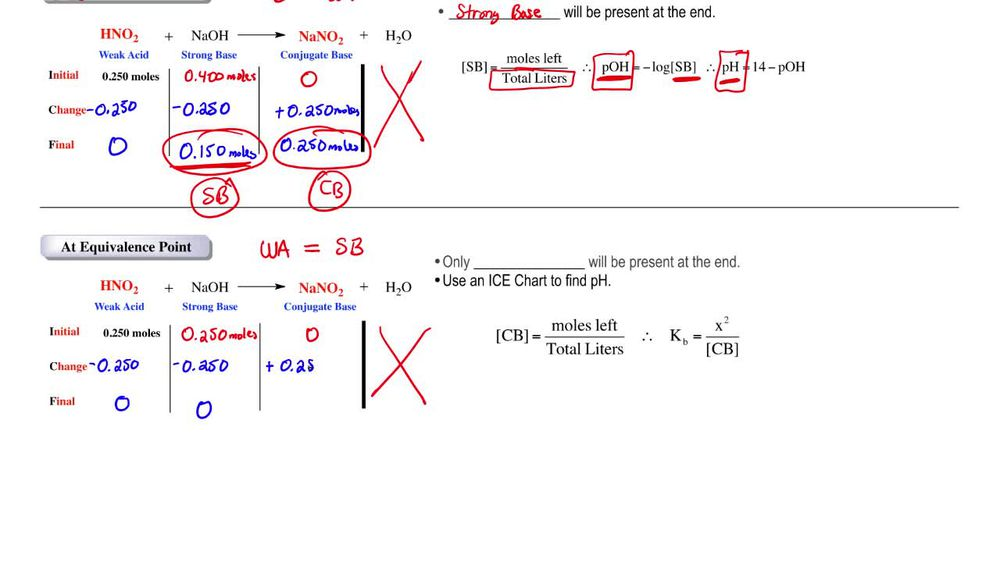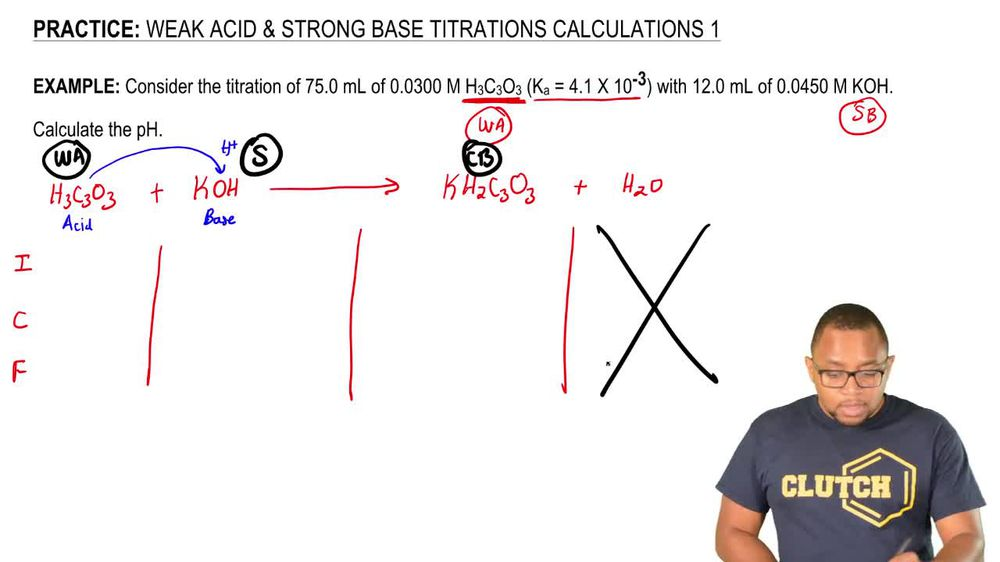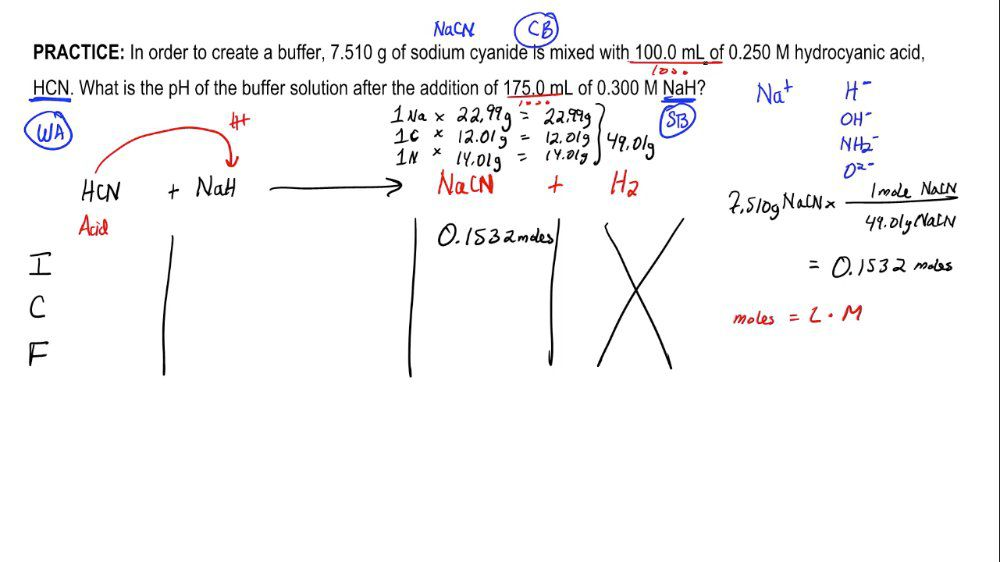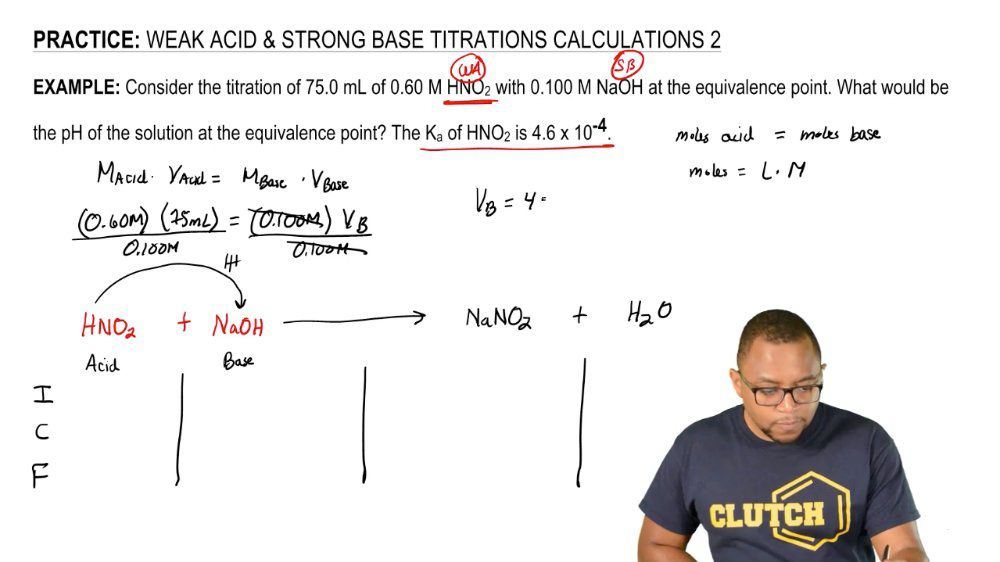Start typing, then use the up and down arrows to select an option from the list.
1. 18. Aqueous Equilibrium2. Weak Acid Strong Base Titrations# Weak Acid-Strong Base Reactions Concept 2

by Jules Bruno
224 views
5
0
when it comes to a typical acid based hydration, there are basically three places in which we discuss our calculations. Is it before the equivalents point at the equivalents point or after the equivalents point? Now, before the equivalence point has been reached, we would say that our weak species in this case are weak. Acid will be greater in amount than our strong species, which is our strong base. So, for example, if we have 0.250 moles off our nitrous acid are weak acid and let's say 0.100 moles of our strong base initially will say that we have zero of our products now. The difference here is will be looking at the react. Inside, the smaller moles will subtract from the larger moles. So what we have left at the end is zero of my strong base and 00. moles of my weak acid. And when it comes to the law of conservation of mass matters, neither created nor destroyed, it just changes forms. That means that if I lose 0.100 moles of my weak acid, it's not really lost, it gets transformed into moles of my conjugal base. So whatever we lose on the react inside, we gain on the product side here. When it comes to the water, we ignore it just like we would in an ice chart. And then what we have left at the end in terms of before the equivalence point. At the end, we'll have a weak acid remaining and conjugate base. Remember, when we have weak acid conjugate base, what does that mean? That means that we have a buffer. And if we have a buffer, we can utilize the Henderson Hasselbach equation, and that will help me find pH. So in this case, we would do pH equals P K, which is just the negative log of my weak acid nitrous acid. So you look up that k value plus log of the conjugate base, what's left of it, which is 0.100 moles, divided by 0.150 moles of my weak acid. And in that way we get our pH if we're dealing with the calculation before the equivalence point. So remember, when it comes to weak acid based height rations, when we're doing the calculations, it'll put us either before the equivalents point at the equivalents pointer. After the equivalents point in all three situations, we have to approach finding pH in a little bit of a different way. Now that we've seen this type of calculation, click on to the next video and see what happens after the equivalence point.